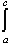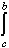#Interactive Real Analysis

Next | Previous | Glossary | Map

## 7.1. Riemann Integral

### Examples 7.1.13(b):

Suppose f(x) = x2 if x1 and f(x) = 3 if x > 1. Findf(x) dx over the interval [-1, 2].
According to part 2 of the previous result we know thatf(x) dx =f(x) dx +f(x) dx
for a < c < b. Let a = -1, c = 1, and b = 2. Then we have:f(x) dx =x2 dx = 1/3 (13 - (-1)3) = 2/3
andf(x) dx =3 dx = 3 (2 - 1) = 3
Thereforef(x) dx = 2/3 + 3 = 11/3
Here we computedx2 dx similarly than in a previous example.
Next | Previous | Glossary | Map